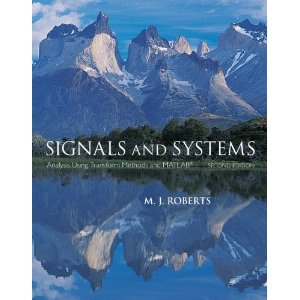## Sunday, September 16, 2012

### Signals and Systems PPT and PDF SLIDESSignals and Systems
Instructor: Akl Robert
Textbook:Signals and Systems: Analysis Using Transform Methods and MATLAB, 2nd edition, M. J. Roberts
Download slides from here

Introduction (Chapter 1 – 1 Lecture), Chapter1.pdf

Mathematical Description of Continuous-Time Signals (Chapter 2 – Lectures), Chapter2.pdf

Continuous-Time Signal Function, Shifting and Scaling

Discrete-Time Signal Description (Chapter 3 – Lectures), Chapter3.pdf

Sinusoids and Singularity Function, Even and Odd Functions

Description of Systems (Chapter 4 – Lectures), Chapter4.pdf

System Modeling - Continuous and Discret

Time-Domain System Analysis (Chapter 5 – Lectures), Chapter5.pdf

Impulse Response,The Convolution Integral

Continuous-Time Fourier Methods (Chapter 6 – Lectures), Chapter6.pdf

Continuous-Time Fourier Series,Continuous-Time Fourier Transform

Discrete-Time Fourier Methods (Chapter 7 – Lectures), Chapter7.pdf

Discrete-Time Fourier Series,Discrete-Time Fourier Transform

The Laplace Transform (Chapter 8 – Lectures), Chapter8.pdf

Development and Properties of the Laplace Transform

The z-Transform (Chapter 9 – Lectures), Chapter9.pdf

Development and Properties of the z-Transform